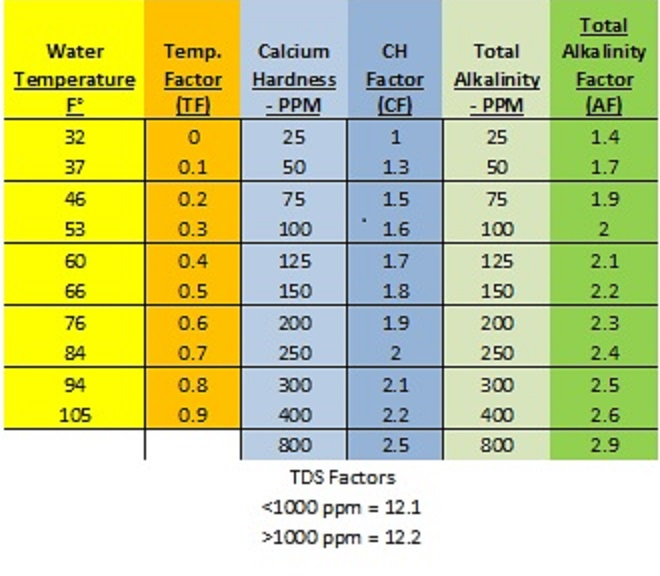# LSI -The Math Explanation For Pool Maintenance

In Blog, Chemistry, Maintenance Tips by Kate Cunha

In my first LSI installment, I wrote a bit about the history and concept of the Langlier Saturation Index. Now it’s time to explore the mathematical equation and some examples, so that you begin to understand how to apply the concept to your own pool maintenance schedule.

First, we must start with the formula and the required factor conversion chart*:

SI = pH +TF + CF + AF – TDSFIdeal: -0.3 -+0.3

*You need the conversion chart to complete the equation. This can be found online (as above) or in the NSPF CPO handbook.

The idea: You start with your pool readings for pH, temperature, calcium hardness, total alkalinity, and TDS. Note that chlorine levels do not play a part in an LSI equation. You then fill out the equation with the appropriate “factors”, found on the chart above. If your test result is not on the chart, use the factor from the next highest number. Complete the equation to find your water’s LSI.

Example 1: Your pool water test readings are as follows: pH 7.8, TA 50, CH 100, Temperature 84°f, TDS 3500

Using the factor conversion chart above, our formula works out to:

7.8 (pH has no conversion factor) + 0.7 (TF) + 1.6 (CF) + 1.7 (AF) – 12.2 (TDSF) = -0.4

In this example, this pool falls outside of the ideal -0.3 to +0.3 ranges and onto the corrosive side of the index. If the water is left as is, the pool could experience pitting of grout and plaster walls, metal erosion, etc.

To balance this pool, we should aim to solve the LSI calculation for 0, or as close to 0 as possible. In this example, the pH is above ideal range, while total alkalinity and calcium hardness are below. If we start by adjusting total alkalinity to 100ppm, lowering the pH to 7.4, and raising the calcium hardness levels to 250ppm, we just may come closer to our goal.

7.4 + .7 (TF) + 2.0 (CF) + 2.0 (TF) – 12.2 (TDSF) = -0.1

Adjust calcium hardness in this pool to 300ppm and you can achieve a LSI balance of 0. When making chemical adjustments to the pool water, start with the total alkalinity, followed by pH and then calcium hardness. Generally temperature is not normally adjusted to reach balance, unless significantly low or high, due to the amount of time and energy required to change the temperature of a pool.

Example 2: pH 7.4, TA 130, CH 150, Temp 103°f, TDS 500.

In this case, our formula works out like this: 7.4 (pH) + .9 (TF) + 1.8 (CF) + 2.2 (AF) – 12.1 (TDSF) = 0.2

This result is within the -0.3 to 0.3 acceptable range, so this water would be considered balanced on the LSI scale. The pool operator would not necessarily need to make any changes to this water, but since the Total Alkalinity is above the ideal range of 80-120, it would make sense to bring the TA down. If the operator adjusts TA to 100, the LSI equation for this pool becomes:

7.4 + .9 (TF) + 1.8 (CF) + 2.0 (AF) -12.1 = 0

Due to the fact that this is a hot body of water, the Calcium Hardness level of 150 is appropriate, and the only adjustment needed to reach a perfect 0 was lowering the Total Alkalinity.

The National Swimming Pool Foundation’s CPO® handbook does an excellent job of explaining the LSI calculation, along with providing numerous examples and the necessary factor conversion chart. If you have access to the CPO® handbook, I highly recommend reviewing the LSI section. In lieu of the book, there are a number of apps and websites that can help.  Whichever route you take, you should be calculating your pool’s LSI once a week and making adjustments, if necessary, to maintain your water in balance. Keeping an eye on the LSI balance of your water on a regular basis will prevent the water in your pool from turning into a corrosive or scaling environment and will save your plaster, your equipment, and your time in the long run.

In the final installment of this look at LSI, we’ll look at a few measures you can take to help ensure that your water always falls within the ideal LSI range, therefore reducing the amount of work needed to maintain clear, safe, balanced water.